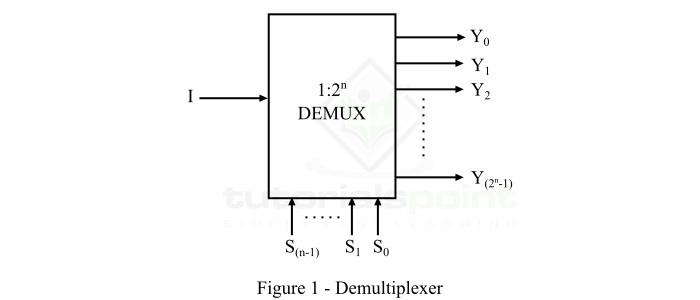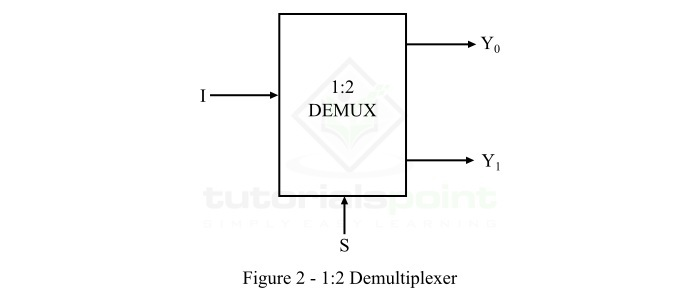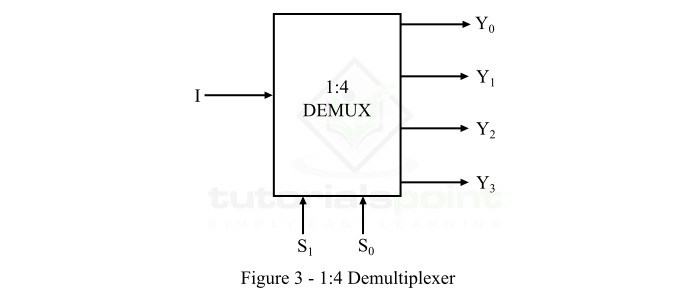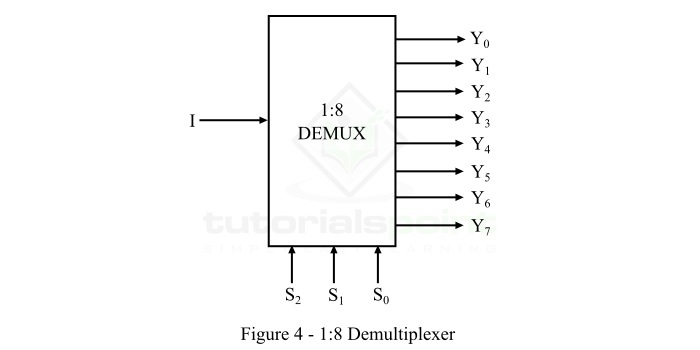# Demultiplexers and Their Applications

## What is a Demultiplexer?

A demultiplexer is a combinational logic circuit that accepts a single input and distributes it over several output lines. Demultiplexer is also termed as DEMUX in short. As demultiplexer is used to transmit the same data to different destinations, hence it is also known as data distributor.

There is another combinational logic circuit named multiplexer which performs opposite operation of the demultiplexer, i.e. accepts several inputs and transmits one of them at time to the output line.

From the definition, we can state that a demultiplexer is a 1-to-2n device. The functional block diagram of a typical 1:2n demultiplexer is shown in Figure-1.It can be seen that the demultiplexer has only one data input line, 2n output lines, and n select lines. The logic level applied to select lines of the demultiplexer determines the output channel to which the input data will be transmitted.

Demultiplexer circuit are the combinational logic circuit widely used in digital decoders and Boolean function generator circuits.

## Types of Demultiplexers

Based on the number of output lines (2n), demultiplexers can be classified into several types. Some commonly used types of demultiplexers are −

• 1:2 Demultiplexer

• 1:4 Demultiplexer

• 1:8 Demultiplexer

Now, let us briefly discuss each type of demultiplexer.

### 1:2 Demultiplexer

The functional block diagram of a 1:2 demultiplexer is shown in Figure-2.The 1:2 demultiplexer consists of 1 input line (I), 1 select line (S), and 2 output lines (Y0 and Y1). The logic level applied at the select line determines the output line to which the input data will be transmitted.

The operation of the 1:2 demultiplexer can be analyzed with the help of its function table given below.

Select Line Outputs
S Y1 Y0
0 0 I
1 I 0

From this function table of 1:2 demultiplexer, we can directly derive the Boolean expression for each output as follow.

$$Y_{0}=\bar{S}I$$

And,

$$Y_{1}=SI$$

### 1:4 Demultiplexer

The functional block diagram of 1:4 demultiplexer is shown in Figure-3.The 1:4 demultiplexer has 1 input line (I), 2 select line (S0 and S1), and 4 output lines (Y0, Y1, Y2, and Y3). The logic level applied to the select lines determines the output line to which the input data (I) will be transmitted.

The operation of the 1:4 demultiplexer can be understood with the help of its function table given below.

Select Line Outputs
S1S0Y3Y2Y1Y0
00000I
0100I0
100I00
11I000

From this truth table of 1:4 demultiplexer, we can directly write the Boolean expression for each output as follow.

$$Y_{0}=\bar{S_{1}}\bar{S_{0}}I$$

$$Y_{1}=\bar{S_{1}}S_{0}I$$

$$Y_{2}=S_{1}\bar{S_{0}}I$$

$$Y_{3}=S_{1}S_{0}I$$

### 1:8 Demultiplexer

The functional block diagram of 1:8 demultiplexer is shown in Figure-4.The 1:8 demultiplexer has 1 input line (I), 3 select line (S0, S1, and S2), and 8 output lines (Y0, Y1, Y2, Y3, Y4, Y5, Y6, and Y7). The logic level applied to the select lines will determine the output line to which the input data (I) will be transmitted.

The operation of this 1:8 demultiplexer can be understood with the help of its function table given below.

Select Line Outputs
S2S1S0Y7Y6Y5Y4Y3Y2Y1Y0
0000000000I
001000000I0
01000000I00
0110000I000
100000I0000
10100I00000
1100I000000
111I0000000

Using the above function table of 1:8 demultiplexer, we can write the Boolean expression for each output as follow.

$$Y_{0}=\bar{S_{2}}\bar{S_{1}}\bar{S_{0}}I$$

$$Y_{1}=\bar{S_{2}}\bar{S_{1}}S_{0}I$$

$$Y_{2}=\bar{S_{2}}S_{1}\bar{S_{0}}I$$

$$Y_{3}=\bar{S_{2}}S_{1}S_{0}I$$

$$Y_{4}=S_{2}\bar{S_{1}}\bar{S_{0}}I$$

$$Y_{5}=S_{2}\bar{S_{1}}S_{0}I$$

$$Y_{6}=S_{2}S_{1}\bar{S_{0}}I$$

$$Y_{7}=S_{2}S_{1}S_{0}I$$

## Integrated Circuits (ICs) Working as Demultiplexer

Demultiplexers can also be built in the form of ICs. There are several types of ICs available that work as demultiplexer. Some common of them are listed below −

• 74139 IC works as a 1:4 Demultiplexer

• 74237 IC works as a 1:8 Demultiplexer

• 74154 IC works as a 1:16 Demultiplexer

The important advantages of demultiplexers are given below −

• By using demultiplexers, we can increase the efficiency of the communication systems.

• Demultiplexer can separate different signals from a mixed signal stream.

• Demultiplexer can decode the signals produced by a multiplexer.

The major disadvantages of demultiplexers are listed below −

• The use of demultiplexer can cause wastage of bandwidth.

• The synchronization of signals can create a delay in the system.

## Applications of Demultiplexers

Demultiplexer is a crucial combinational logic circuit which is used in a number of applications. Some important uses of demultiplexers are listed below −

• Demultiplexers are used in several input and output devices for data routing.

• Demultiplexers are used in digital control systems to select one signal from a mutual stream of signals.

• Demultiplexers are also employed for data transmission in synchronous systems.

• Demultiplexers are also utilized in data acquisition systems.

• Demultiplexers can be used for generating Boolean functions.

• Demultiplexers can be used in serial to parallel converters.

• Demultiplexers are used for broadcasting of ATM packets.

• Demultiplexers can also be used to design automatic test equipment, etc.

This is all about demultiplexer, its types, and applications.

Updated on: 19-Apr-2023

2K+ Views